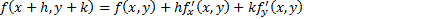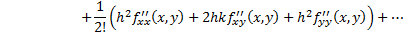/

### Enterprise Risk Management Formula Book 2. Series expansions (for real-valued functions)

2.1          Exponential function and natural logarithm (log) function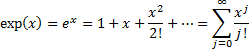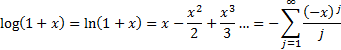2.2          Binomial expansion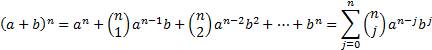where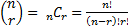is the binomial coefficient.

If we substitute into the binomial expansion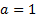,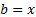and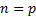we have (converges for any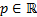if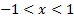):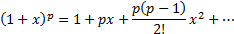A corollary is that: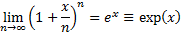2.3          Taylor series expansion

For one variable: if series converges (where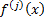is the’th derivative of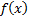and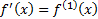,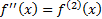etc.):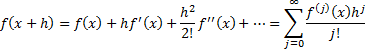For more than one variable: e.g. for two variables, if series converges (where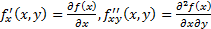etc.):